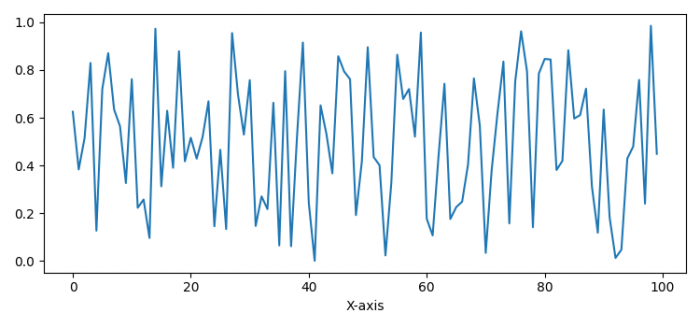# How to access axis label object in Matplotlib?

To axes axis label object in Matplotlib, we can use ax.xaxis.get_label().get_text() method.

## Steps

• Set the figure size and adjust the padding between and around the subplots.
• Create a figure and a set of subplots.
• Initialize a variable, N, for number samples.
• Create random data points using numpy.
• Plot x data points using plot() method.
• Set X-axis label using set_xlabel() method.
• To get the xlabel, use get_label() method and get_text() method.
• To display the figure, use show() method.

## Example

import numpy as np
import matplotlib.pyplot as plt

plt.rcParams["figure.figsize"] = [7.50, 3.50]
plt.rcParams["figure.autolayout"] = True

fig, ax = plt.subplots()
N = 100
x = np.random.rand(N)
ax.plot(x)
ax.set_xlabel("X-axis")
x_lab = ax.xaxis.get_label()
print("Label is: ", x_lab.get_text())

plt.show()

## Output